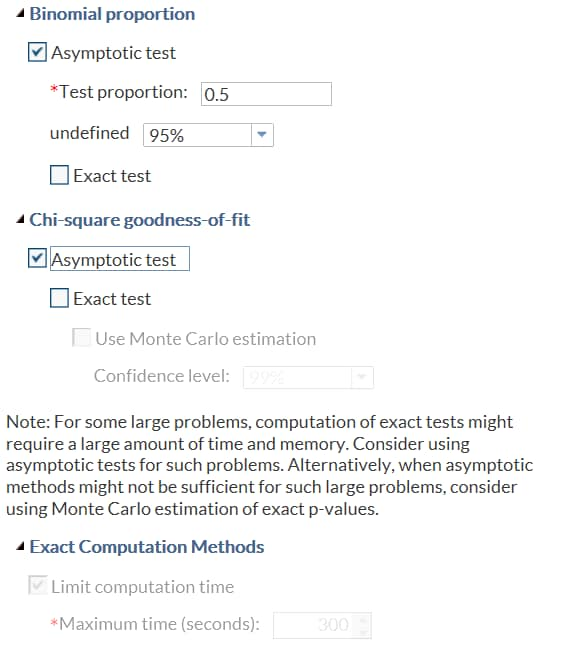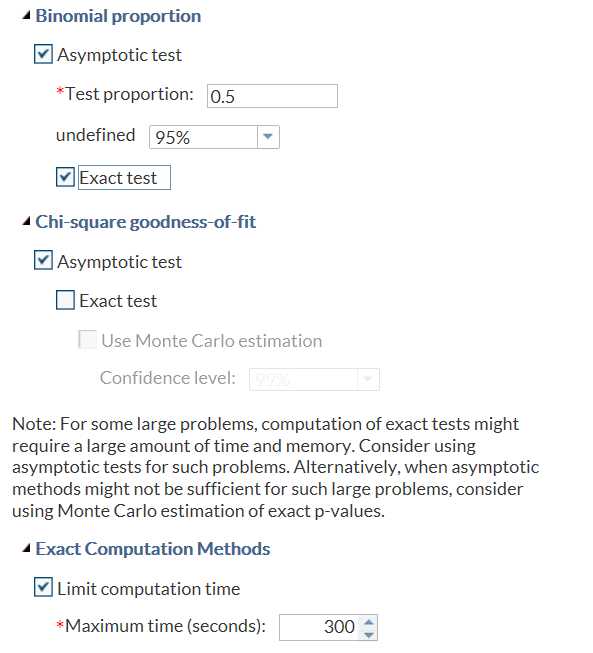# Example 6: Compound Condition Using AND and OR Logic

In this example from the One-Way Frequencies task, the Maximum time (seconds) option is available only if you select the Exact test check box (for Binomial proportion or Chi-square goodness of fit) and the Limit computation time check box. (In the Tasks section, the One-Way Frequencies task is in the Statistics group.)
In this example, SECONDS is the name of the number stepper control. chkEXACTP is the name of the Exact test check box for binomial proportions. chkCHIEXACTP is the name of the Exact test check box for chi-square goodness of fit. chkLIMIT is the name of the Limit computation time check box.
```<Option name="chkEXACTP" indent="1" defaultValue="0"
inputType="checkbox">Exact test</Option>
<Option name="chkCHIEXACTP" indent="1" defaultValue="0"
inputType="checkbox">Exact test</Option>
<Option name="chkLIMIT" indent="1" defaultValue="1"
inputType="checkbox">Limit computation time</Option>
<Option name="SECONDS" indent="2" defaultValue="900"
inputType="checkbox">Maximum time (seconds):</Option>

<Dependency condition="(((\$chkEXACTP=='1') || (\$chkCHIEXACTP=='1'))
&amp;&amp; (\$chkLIMIT=='1'))"/>
<Target conditionResult="true" option="SECONDS" action"enable"/>
<Target conditionResult="false" option="SECONDS" action"disable"/>
</Dependency>```
By default, neither of the Exact test check boxes is selected, so the Maximum time (seconds) option is not available. Here is the result that appears on the Options tab:When you select either of the Exact test check boxes and the Limit computation time check box, the Maximum time (seconds) option is available. Here is the result that appears on the Options tab: